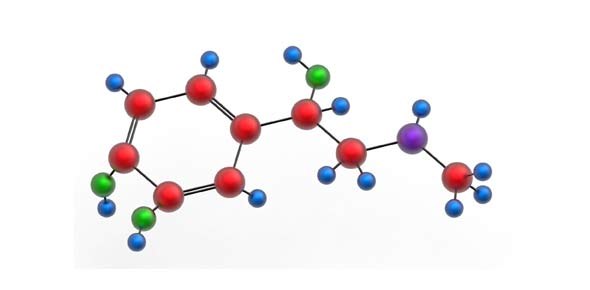# Unit 8 - Chemical Compound Review

25 Questions | Total Attempts: 62SettingsWe have just finished covering unit 8 in class today. The simple quiz below is set to help you review what you understood about chemical compounds, their formation, appearance and reaction. Give it a go and share your scores in the comment section. Best of luck as you tackle it.

Related Topics
• 1.
A _____ is a large moelcule made of carbon and hydrogen atoms.
• A.

Hydrocarbon

• B.

Solute

• C.

Hydrogen oxide

• D.

Solvent

• 2.
Adding a solute to a solvent _____ the freezing point of a solvent.
• A.

Lowers

• B.

Raises

• C.

Does not change

• D.

Doubles

• 3.
Air contains 78 percent nitrogen, 21 percent oxygen, and one percent argon. Which gas is the solvent?
• A.

Argon

• B.

None of the answers are correct

• C.

Nitrogen

• D.

Oxygen

• 4.
What characteristic of water makes it the universal solvent?
• A.

Nonpolar

• B.

Large molecules

• C.

Long-chain hydrocarbon

• D.

Polar

• 5.
Why is it dangerous to take large doses of some nonpolar vitamins?
• A.

They are water soluble and become concentrated in body tissues.

• B.

These substances cannot be utilized by the body.

• C.

They are fat soluble and accumulate in body tissues.

• D.

They cannot dissolve in fat and instead dissolve in the water inside body cells.

• 6.
Gases are _____ in cooler solvents.
• A.

Insoluble

• B.

Less soluble

• C.

More soluble

• D.

Precipitates

• 7.
Which attributes enables water molecules to dissolve sugar?
• A.

Nonpolarity of water

• B.

Liquid state of the solute

• C.

Stationary nature of water molecules

• D.

Electrical charge

• 8.
Sterling silver contains 92.5 percent silver and 7.5 percent copper. Which substance is the solute?
• A.

Solids do not form solutions

• B.

Platinum

• C.

Silver

• D.

Copper

• 9.
Because electrons are distributed equally, a hydrocarbon is ____.
• A.

Insoluble

• B.

Nonpolar

• C.

Polar

• D.

Nontoxic

• 10.
Vitamin C is a(n) _____ compound and dissolves readily in water.
• A.

Polar

• B.

Oily

• C.

Nonpolar

• D.

Fat soluble

• 11.
Which of the following statements is true?
• A.

Nonpolar solvents are useful for dissolving polar solutes.

• B.

Polar solvents are useful for dissolving nonpolar solutes.

• C.

Nonpolar solvents are not useful because they do not form solutions with water.

• D.

Nonpolar solvents are useful for dissolving nonpolar solutes.

• 12.
Which of the following is the most precise term?
• A.

Supersweet

• B.

Dilute

• C.

40 percent juice by volume

• D.

Concentrated

• 13.
Which of the following increases rate of evaporation?
• A.

Decreasing surrounding temperature

• B.

Increasing attraction between solvent moelcules

• C.

Combining polar solvents with polar solutes

• D.

Decreasing attraction between solvent molcules

• 14.
The process in which water molecules draws ions away from a crystalline solid and into solution is _____.
• A.

Supersaturation

• B.

Nonpolarization

• C.

Concentration

• D.

Dissociation

• 15.
Which of the actions increases the rate of dissolving?
• A.

Stirring the solution

• B.

Decreasing the pressure

• C.

Using larger-sized crystals

• D.

Decreasing the temperature

• 16.
A mixture that appears to have the same composition, color, and density and is mixed at the molecular level is called a(n) ____.
• A.

Element

• B.

Suspension

• C.

Solution

• D.

Heterogenous mixture

• 17.
_____ is the maximum amount of a solute that can be dissolved in a given amount of solvent at a given temperature.
• A.

Polarity

• B.

Concentration

• C.

Supersaturation

• D.

Solubility

• 18.
A solution that contains all the solute it can hold at a given temperature is _____.
• A.

Unsaturated

• B.

Supersaturated

• C.

Soluble

• D.

Saturated

• 19.
____ are compounds that form charged particles.
• A.

Nonpolar components

• B.

Ions

• C.

Electrolytes

• D.

Nonelectrolytes

• 20.
Which of the following is a solution?
• A.

Muddy water

• B.

Chlorine

• C.

Milk

• D.

Salt water